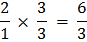### Sample Problem

Complete the ratio table.

 2 1 4 2 6 8 4

#### Solution

Equivalent fractions represent equivalent ratios.

Then, start with 2:1. Write the ratio as a fraction. Multiply the numerator and denominator by the same number to find an equivalent ratio.Thus, the missing number is 3.